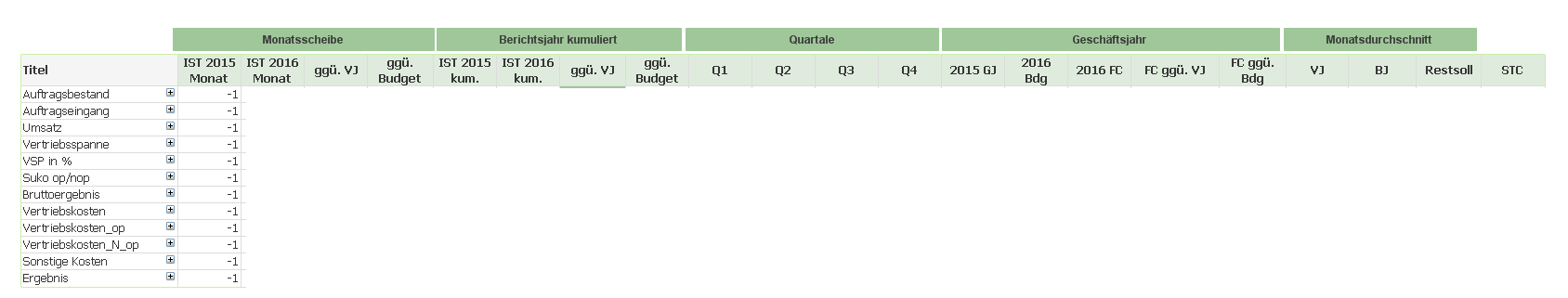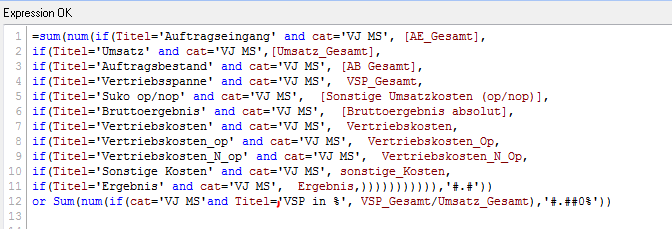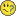# QlikView Scripting

Discussion Board for collaboration on QlikView Scripting.

Announcements
QlikWorld, June 24-25, 2020. Free virtual event for DI and DA gurus. Register Today
cancel
Showing results for
Did you mean:
HighlightedContributor II

## Too many formulas, HELP

Hello QlikView Community,

I am really stuck here and I hope, you can help me out.

So as you can see in the pictures I am trying to make an pivot table, that has all the relevant numbers of an bussines like new orders (Auftragseingang), sales(Umsatz) and profit(Ergebnis). Relative to the name in the first column I want to see the different numbers for every row. So for example second row new orders(Auftragseingang). Thats what the if-function "title" is for. Also in every column there are different numbers. For example last year, this year or budget. This is what the "cat='VJ MS'" is for.(Last years numbers).

Even it is a huge formula it did work out well, until I now also want to see for example profit/sales in percent. This is the last row of the formula and its not working at all. It even destroys all the other numbers. Maybe somebody understood my problem and helps me to make an smaller but more efficient formula?

Thank you so much

Kind regards

TinaTags (2)
8 Replies
HighlightedMVP

## Re: Too many formulas, HELP

Would you be able to provide sample

Before develop something, think If placed (The Right information | To the right people | At the Right time | In the Right place | With the Right context)
HighlightedMVP

## Re: Too many formulas, HELP

I agree with Anil, I think we would need to look at a sample before we can propose anythingHighlightedContributor II

## Re: Too many formulas, HELP

Sum(num(if(cat='Last years numbers (VJ MS)'and Titel='profit in %' ,total_profit/sales),'#.##0%'))

Does that help ? In the same forumla I also want to see profit in normal numbers,

The expression would be then:

sum(if(Titel='Profit' and cat='Last years numbers (VJ MS)', total_profit))

Thank you!

HighlightedSpecialist II

## Re: Too many formulas, HELP

This is indeed a rather frustrating problem with P&L and similar reports.

My recommended approach is to create a mapping file (I use Excel) with the names of the rows you want in the dimension and the actual data they should have, e.g.

 PNL_NUM PNL_NAME CATEGORY PNL_STYLE PNL_ISPERCENT 10 Total Sales Sales 0 0 20 Total Cogs Cogs 0 0 25 Gross Profit Sales 1 0 25 Gross Profit Cogs 1 0 29 % GP Sales 2 1 29 % GP Cogs 2 1

Note the addition of a NUM field (to make sure the rows are in the right oder), STYLE (for bolding / backgrounds), and ISPERCENT (I use this to determine whether a row is a sum or a percent-of-sales value). CATEGORY is the actual data that should be associated with my synthetic (PNL_) rows.

Your formula would then be something along the lines of (I'm freehand writing this, so it may not be entirely accurate):

If(PNL_ISPERCENT=0,Num(Sum(VALUE),'#,##0'),

Num( Sum(VALUE) / Sum({< PNL_NAME = {"Total Sales"} >} Total VALUE),'#,##0.0%'))

The Num() is necessary to format the expression as a percent or a number depending on the line type. The first half of the formula deals with regular sum values while the latter deals with percent values (sum of the current row - which may contain multiple categories - divided by total sales in my example, but your situation may differ).

Unfortunately - and this is where it starts getting ugly most of the time - these reports tend to have a large number of expressions (in your example you're showing 17 of them), and each requires additional set analysis to get the correct data - so you'll have to adapt the base formula for each expression.

I hope this helps - I am not able to include my sample file as scrambling the data without breaking the actual example would be very time-consuming.

HighlightedMVP & Luminary

## Re: Too many formulas, HELP

This couldn't work then you concat an expression with an OR and another expression which led to an error. I suggest to change the expression in this way:

if(cat = 'VJ MS',

pick(match(Title, 'Umsatz', 'Auftragsbestand', ....),

num(sum([Umsatz_Gesamt]), '#.#'),

num(sum([AB Gesamt]), '#.##0,0%'), // it's the wrong format for this expression - here only as example

....))

- Marcus

HighlightedMVP

## Re: Too many formulas, HELP

To me it seems that your formula is broken. Check these two initial anomalies:

• Your last IF() function in the first Sum() has space for a third parameter, but the parameter itself is missing. That makes the expression illegal.
• A Sum() function produces a numerical value, and as such isn't entirely reliable to operate in logical evaluations. IMHO
Sum() or Sum() may not always work out as expected.

A better expression would be:

= IF (cat = 'VJ MS',

Pick(Match( 'VSP in %', 'Auftragseingang', usw ...)

num(Sum(VSP_Gesamt) / Sum(Umsatz_Gesamt), '#.##0%'),

num(Sum(AE_Gesamt), '#.#'),

usw...

)

)

Best,

Peter

HighlightedMVP & Luminary

## Re: Too many formulas, HELP

Did you try the suggestion from Peter or me?

- Marcus

HighlightedContributor II

## Re: Too many formulas, HELP

Thank you, for all your answers!I tried a lot, but none of them worked....

I think the problem is, that expressions like 'Auftragseingang', VSP in % and all the titels are only there, so the programm can match it with the first column of the table, and select the right data for each row.

But maybe I am just misunderstanding something.

I tried somethin else (it is even more complex) and now only the number format is wrong.

The percentage shows 0,038% but it should show 38% ??

The problem is, that I cant show you the table because its confidential=num(sum(if(Titel='Auftragseingang' and cat='VJ MS', [AE_Gesamt],

if(Titel='Umsatz' and cat='VJ MS',[Umsatz_Gesamt],

if(Titel='Auftragsbestand' and cat='VJ MS', [AB Gesamt],

etc......

)))))), '#.###,0')

&

num(

(sum(if(cat='VJ MS'and Titel='VSP in %', VSP_Gesamt,

if(cat='VJ MS'and Titel='Bruttoergebnis in %', [Bruttoergebnis absolut],

etc.....

)))

/

(sum(if(cat='VJ MS' and Titel='VSP in %', Umsatz_Gesamt,

if(cat='VJ MS' and Titel='Bruttoergebnis in %', Umsatz_Gesamt,

etc....

))),'#.##0%')

Thank you for trying to help me!